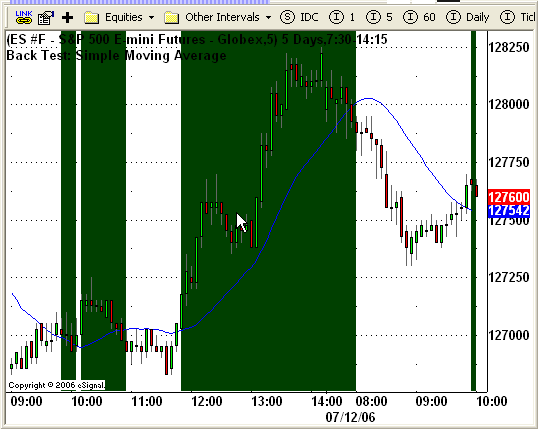# BtSimpleMA.efs

ICE Data Services -

 BtSimpleMA.efs EFSLibrary - Discussion Board

File Name: BtSimpleMA.efs

Description:
This formula is an introductory example of how to code a back testing strategy.

Formula Parameters:
NA

Notes:
This formula is a back testing formula to be used in conjunction with the Strategy Analyzer. It is not intended for real time analysis. This formula requires version 7.9.1 or later.

The following strategy has a very simple set of rules and will only take long positions. A long position will be taken when a bar closes above a 21-period simple moving average. The position will be closed when a subsequent bar closes below the moving average.`/*********************************Copyright ) eSignal, a division of Interactive Data Corporation. 2006. All rights reserved. This sample eSignal Formula Script (EFS) may be modified and saved under a new filename; however, eSignal is no longer responsible for the functionality once modified.eSignal reserves the right to modify and overwrite this EFS file with each new release.This sample is for educational purposes only. It is not intended for trading.Strategy Logic: This Strategy example is a basic strategy that only takes long positions when a bar closes above a 21-period simple moving average. The long position will be closed on the next bar that closes below the simple moving average.*********************************/function preMain() { setPriceStudy(true); setStudyTitle("Back Test: Simple Moving Average"); setCursorLabelName("SMA", 0); setDefaultBarFgColor(Color.blue, 0);}// Global Variablesvar xSMA = null;var bInit = false; // initialization flagfunction main() { // Back Testing formulas are not for real time analysis. // Therefore, prevent processing and exit at bar 0. if (getCurrentBarIndex() == 0) return; if(bInit == false) { // This code block executes only once at the beginning of initialization. // Initialize SMA Series Object. xSMA = sma(21); bInit = true; // Prevents this code block from executing again. } // Retrieve previous bar's values of SMA Series for back testing conditions. var nSma = xSMA.getValue(-1); // Validate the study variables to ensure they contain valid data. if(nSma == null) return; // Exit the formula if invalid data. // Long Trade Signal if (Strategy.isLong() == false && close(-1) >= nSma) { /***** If the strategy is not long and the previous bar's close is equal to or greater than the previous bar's SMA, go long at the open of the current bar. *****/ Strategy.doLong("Long Signal", Strategy.MARKET, Strategy.THISBAR); } else if (Strategy.isLong() == true && close(-1) < nSma) { // Long Exit Signal /***** If the strategy is long and the previous bar's close is below the previous bar's SMA, exit the long position at the open of the current bar. *****/ Strategy.doSell("Long Exit Signal", Strategy.MARKET, Strategy.THISBAR); } if(Strategy.isLong()) { setBarBgColor(Color.darkgreen); } // Plot the current bar's SMA values. return xSMA.getValue(0);}`